# Poincare polynomial

This article describes an invariant of topological spaces that depends only on its homology groups

## Definition

Given a topological space$X$ which has finitely generated homology, the Poincare polynomial of$X$ is defined as the generating function of its Betti numbers, viz the polynomial where the coefficient of$x^q$ is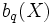$b_q(X)$.

Note that for a space with homology of finite type, all the Betti numbers are well-defined, but infinitely many of them are nonzero, so we get a Poincare series instead of a Poincare polynomial.

The Poincare polynomial of$X$ is denoted$PX$.

## Particular cases

Case for the space Value of Poincare polynomial (note: if the space is not a single space but a parameterized family of spaces, the polynomial may also depend on the parameter) Further information
a contractible space 1
circle$1 + x$ homology of spheres
torus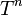$T^n$ (product of$n$ copies of the circle)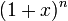$(1 + x)^n$ homology of torus
sphere$S^n$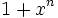$1 + x^n$ homology of spheres
product of spheres$S^{m_1} \times S^{m_2} \times \dots \times S^{m_r}$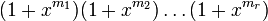$(1 + x^{m_1})(1 + x^{m_2}) \dots (1 + x^{m_r})$ homology of product of spheres
compact orientable genus$g$ surface$1 + 2gx + x^2$ homology of compact orientable surfaces
real projective plane$\R\mathbb{P}^2$ 1 homology of real projective space
even-dimensional real projective space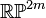$\R\mathbb{P}^{2m}$ 1 homology of real projective space
odd-dimensional real projective space$\R\mathbb{P}^n$,$n = 2m + 1$$1 + x^n$ homology of real projective space
complex projective space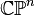$\mathbb{C}\mathbb{P}^n$$1 + x^2 + \dots + x^{2n}$ homology of complex projective space

## Facts

### Disjoint union

Further information: Poincare polynomial of disjoint union is sum of Poincare polynomials

The Poincare polynomial of a disjoint is the sum of the Poincare polynomials of the individual spaces: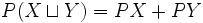$P(X \sqcup Y) = PX + PY$

### Wedge sum

The Poincare polynomial of a wedge sum of two path-connected spaces, is the sum of their polynomials minus 1.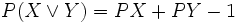$P(X \vee Y) = PX + PY - 1$

### Product

Further information: Poincare polynomial of product is product of Poincare polynomials

The Poincare polynomial of the product of the spaces is the product of the Poincare polynomials. This is a corollary of the Kunneth formula (note that we are assuming that both spaces have finitely generated homology).

A particular case of this (which can be proved directly using the exact sequence for join and product and does not require appeal to the Kunneth formula) is: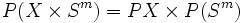$P(X \times S^m) = PX \times P(S^m)$